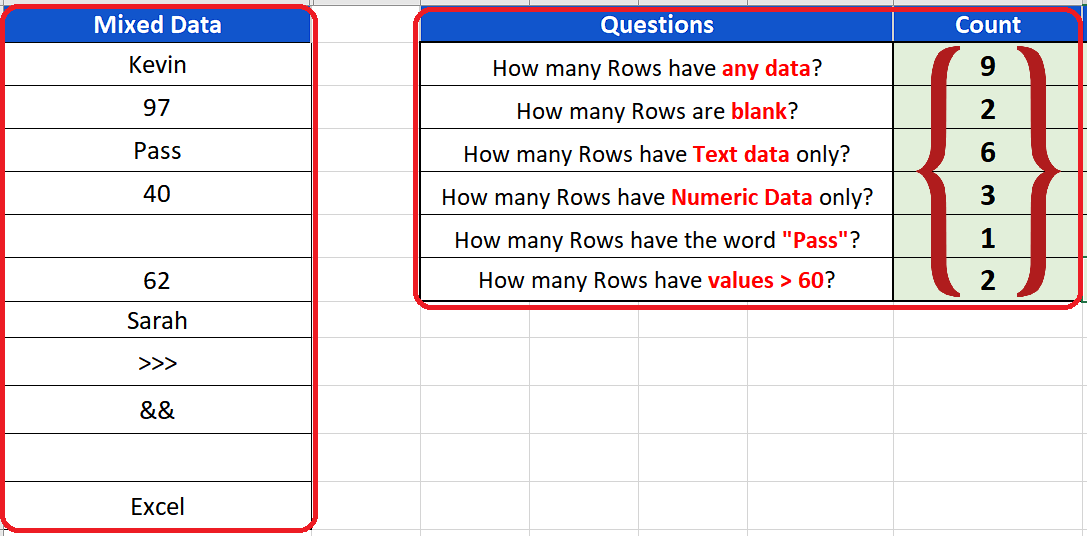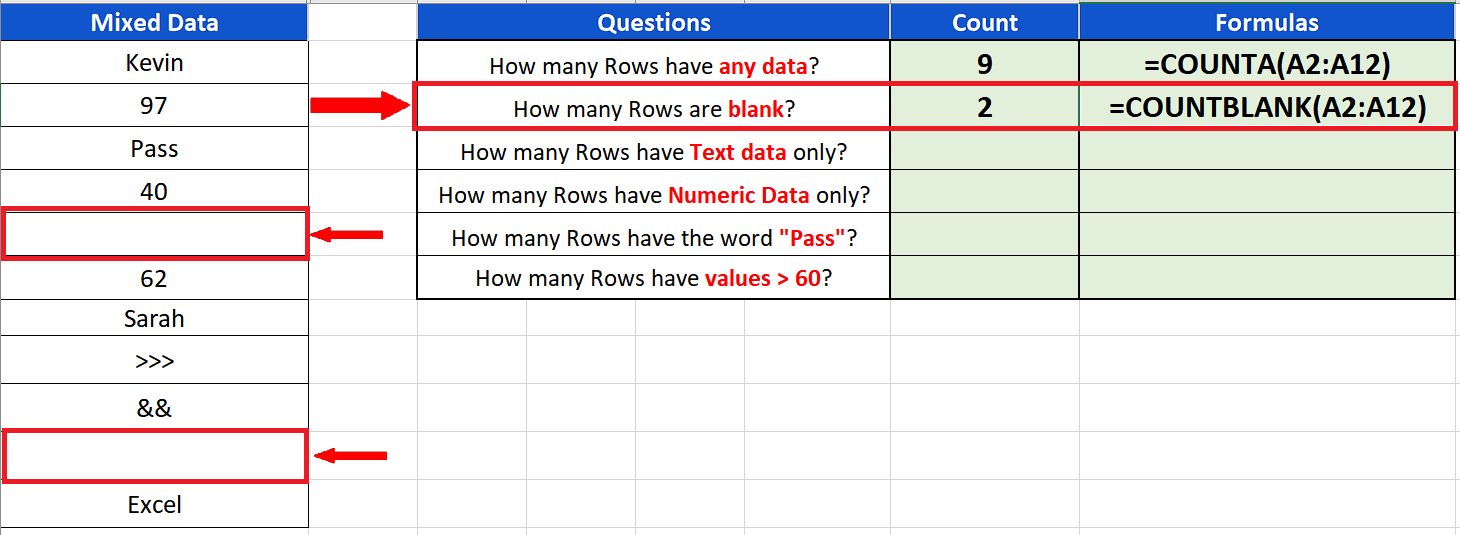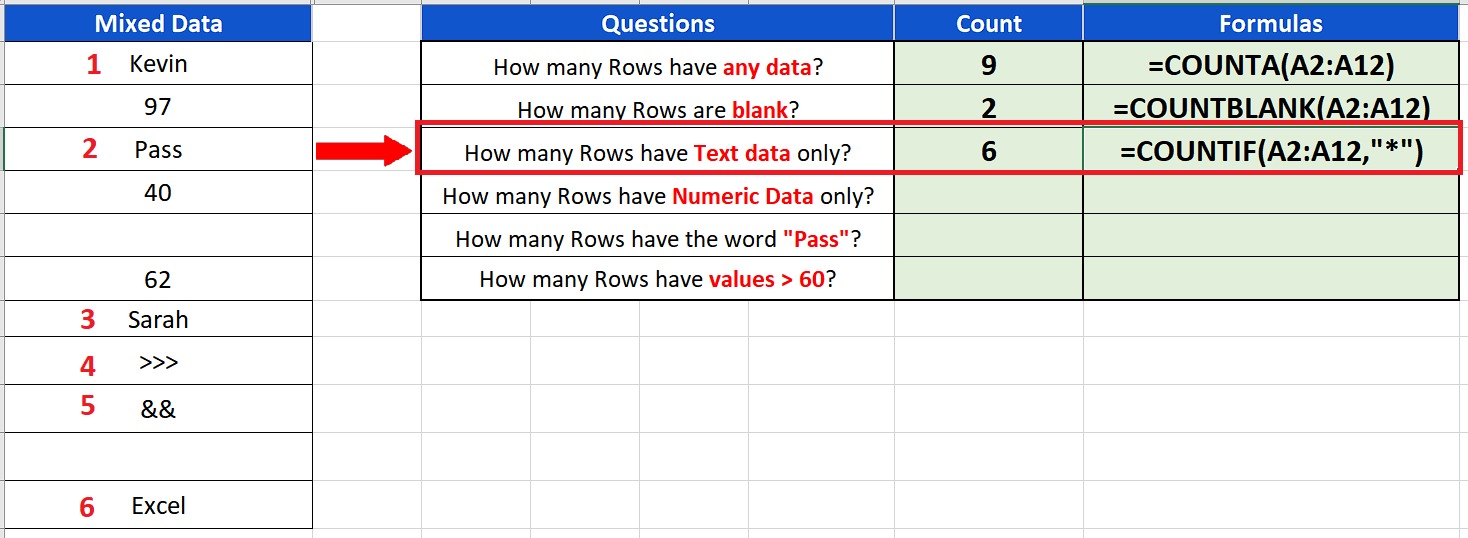# How to count rows in Excel

Sometimes when you are working with big data, you need to know the number of rows in your data set, based on specific conditions. So in today’s tutorial we are going to learn how to count the number of rows in your data set having some key features.

Consider the following data set,

In this data set we’ll learn various ways to find out the number of rows in the data set based upon different conditions.

• Count rows with any data
• Count rows with blanks
• Count rows with text only
• Count rows with numeric data only
• Count rows with a specific word in it
• Count rows fulfilling a logical comparison condition

So let’s dive into the data and learn how we can use simple formulas to count the rows step by step.Excel is a very powerful software when it comes to performing data analysis, simple mathematical, statistical or financial calculations. It comes up with a lot of functions which help us in doing the daily tasks.

### Step 1 – Count rows with any type of data– We can count the rows which have any type of data with a simple formula

=COUNTA(A2:A12)

COUNTA will count all those rows which have any data in it except the blanks. As we can see, our data set had 9 rows with some data in it. So the result is 9.

### Step 2 – Count rows which are blank– We can count the rows which have any type of data with a simple formula

=COUNTBLANK(A2:A12)

COUNTBLANK will count the rows only which are blank in the given range. As we have only two blanks so the desired result is 2.

### Step 3 – Count rows with text data only– We can count the rows which have any type of data with a simple formula

=COUNTIF(A2:A12, “*”)

COUNTIF with wildcard “*” will count only those rows which have text data in it. As we can see, our data set had 6 rows with text data in it. So the result is 6.

### Step 4 – Count rows numeric data only– We can count the rows which have any type of data with a simple formula

=COUNT(A2:A12)

COUNT will count the rows only which have numeric data in them within the given range of data. As we have only three such rows so the desired result is 3.

### Step 5 – Count rows with specific text– We can count the rows which have any type of data with a simple formula

=COUNTIF(A2:A12, “Pass”)

COUNTIF with special keyword “Pass” will count only those rows which have the exact word “Pass” in it. As we can see, our dataset had only 1 row1 with “Pass” in it. So the result is 1.

### Step 6 – Count rows meeting a logical condition– We can count the rows which have any type of data with a simple formula

=COUNTIF(A2:A12, “>60”)

COUNTIF with special condition “>60” will count the rows only which have numeric values greater than 60 within the given range of data. As we have only two such rows so the desired result is 2.